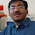### Divisibility of 111...1111

Source: Asked to Russian 7th Graders - Taken from Wild For Math Blog

Problem:
Is it true that among the numbers consisting of only "1"s (1; 11; 111; 1111; etc.) there is a number (maybe many) that is divisible by 572,003?

Here 572,003 is taken arbitrarily. Is it true for all numbers?

Update (November 02, 2011):
Solution posted in comments by NG a.k.a Nikhil Garg (IITD CSE Senior Undergraduate), Rudradev Basak (IITD CSE Senior Undergraduate, IMO Bronze Medalist), Yashoteja Prabhu (RA at Microsoft Research, IITB CSE 2011 Alumnus), Siva, Garvit Juniwal (IITB CSE Senior Undergraduate)! Thanks

1.It is true for all numbers that are coprime to 10 i.e not divisible by either 2 or 5.
Also clearly any even number won't divide any number in sequence as last digit of each of these is odd.
Similarly any number divisible by 5 wont divide any of the numbers in sequence as last digit is not one of 0/5

Proof :
Let number be N. Take first N terms of the sequence 1, 11, 111, ...
If one of these is divisible by N then we are done. Else none of them is 0 mod N. So all the values mod N are from 1 .. N-1. These are only N -1 different values so at least two numbers in sequence would have collided at some residue.
Say 111...(a times) and 111...(b times) are both k mod N.
Consider their difference. It would be a number of form some 1s followed by some 0s. And this would be 0 mod N. As N was coprime to 10, we can divide by 10 to get a number of form 111.. divisible by N.

2.Yes. In general this is true for all numbers n which are not divisible by 2 or 5 (or gcd(n,10)=1 )
Denote (10^i-1)/9 by F(i)
Proof: For a given n, lets write down each number in the series F(i) modulo n. Since this is an infinite series some two remainders are the same. Let these correspond to F(a) and F(b), with a<b. Then this means n divides the difference of these two, which equals F(b)-F(a) = F(b-a)*10^a. Since gcd(n,10) = 1, so n divides F(b-a)

3.Let N = 572003

Take first N integers of the form 1111....

Look at the remainders when each of these numbers is divided by N.
Either one of them is '0' (in which case N divides that number) or there are two numbers with same remainder.
In second case, take the difference of those two numbers(A & B).

A-B = 1111... * 10^x

Now, N=89x6427. So N does not have a common factor with 10^x.
=> N|1111... which is in fact contradiction of initial assumption.

This is true for all numbers not having 2 or 5 as a factor, proving along the same lines as above.
And if a number has 2 or 5 as a factor, it cannot divide 11111....

4.By Euler's Theorem all numbers that are coprime to 2 and 5 divide a number that is composed of only 1's

to obtain such a number one can use Euler's Totient function but we maynot get the smallest such number.

5.Let us try to find a number of the form 111..111 divisible by some arbitrary number n. Consider the following n+1 numbers:

1
11
111
.
.
.
111...111 (n+1 times)

Some two of them(say a,b where a>b) will be same modulo n. So, a-b is divisible by n and is of the form 111...11000...00. It is clear that if n does not admit either 2 or 5 as a divisor, then a number of the form 111...111 will also be divisible by n, which is true for 572,003.

6.All solutions are correct (essentially the same) :)
Thanks!

7.What about 111 and 7 or 111 and 13?
Both 7 and 13 and many others are not divisible by 10 and they don't divide the given number.

Have I misunderstood the problem?

8.@Unknown, the question is really:

Let the set of all numbers co-prime to 10 be X
Let the set of all numbers of the form 11...111 be Y

For all x belonging to X, there exists a y belonging to Y, such that x | y

Hope that helps!